Search IntMath
Close

450+ Math Lessons written by Math Professors and Teachers

5 Million+ Students Helped Each Year

1200+ Articles Written by Math Educators and Enthusiasts

Simplifying and Teaching Math for Over 23 Years

# 6. Expressing a sin θ ± b cos θ in the form R sin(θ ± α)

by M. Bourne

In electronics, we often get expressions involving the sum of sine and cosine terms. It is more convenient to write such expressions using one single term.

## Our Problem:

Express a sin θ ± b cos θ in the form

R sin(θ ± α),

where a, b, R and α are positive constants.

#### Solution:

First we take the "plus" case, (θ + α), to make things easy.

Let

a sin θ + b cos θR sin (θ + α)

(The symbol " ≡ " means: "is identically equal to")

Using the compound angle formula from before (Sine of the sum of angles),

sin(A + B) = sin A cos B + cos A sin B,

we can expand R sin (θ + α) as follows:

R sin (θ + α)

R (sin θ cos α + cos θ sin α)

R sin θ cos α + R cos θ sin α

So

a sin θ + b cos θ R cos α sin θ + R sin α cos θ

Equating the coefficients of sin θ and cos θ in this identity, we have:

For sin θ:

a = R cos α ..........(1)
(in green above)

For cos θ:

b = R sin α .........(2)
(in red above)

Eq. (2) ÷ Eq.(1):

b/a=(R sin alpha)/(R cos alpha)=tan alpha

So

alpha=arctan\ b/a

(α is a positive acute angle and a and b are positive.)

Now we square each of Eq. (1) and Eq. (2) and add them to find an expression for R.

[Eq. (1)]2 + [Eq. (2)]2:

a2 + b2

= R2 cos2 α + R2 sin2 α

= R2(cos2 α + sin2 α)

= R2

(since cos2 A + sin2 A = 1)

So

R=sqrt(a^2+b^2

(We take only the positive root)

In summary, if

alpha=arctan\ b/a

and

R=sqrt(a^2+b^2)

then we have expressed a sin θ + b cos θ in the form required:

a sin θ + b cos θ = R sin(θ + α)

You will notice that this is very similar to converting rectangular to polar form in Polar form of Complex Numbers. We can get α and R using calculator, similar to the way we did it in the complex numbers section.

### The Minus Case

Similarly, for the minus case, we equate a sin θb cos θ with the expansion of R sin (θ − α) as follows (note the minus signs carefully):

a sin θb cos θ R cos α sin θR sin α cos θ

Once again we will obtain (try it yourself!):

alpha=arctan\ b/a

and

R=sqrt(a^2+b^2)

Our equation for the minus case is:

a sin θb cos θ = R sin(θ − α)

## Equations of the type a sin θ ± b cos θ = c

To solve an equation in the form

a sin θ ± b cos θ = c,

express the LHS in the form R sin(θ ± α) and then solve

R sin(θ ± α) = c.

### Exercises - Sine Form

1. (a) Express   4 sin θ + 3 cos θ  in the form R sin(θ + α).

4 sin θ + 3 cos θ = 2     for 0° ≤ θ < 360°.

#### Part (a)

Firstly, let:

4 sin θ + 3 cos θR sin(θ + α)

Then we need:

R=sqrt(4^2+3^2)=sqrt25=5

alpha=arctan(3/4)=36.87^@

So

4 sin θ + 3 cos θ = 5 sin(θ + 36.87°)

#### What have we done?

The components of the original function were:

(i) 4 sin θ (in black)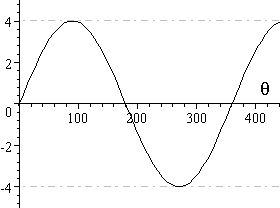(ii) 3 cos θ (in blue, with 4 sin θ)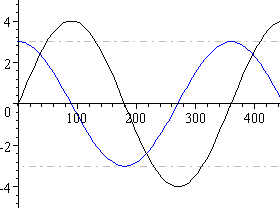When we add these 2 components we get a sine curve that has been shifted to the left by 36.87^@:

4 sin θ + 3 cos θ = 5 sin(θ + 36.87°) (in red)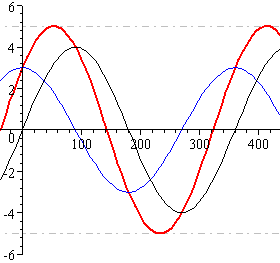#### Part (b)

From part (a),

4 sin θ + 3 cos θ = 5 sin(θ + 36.87°)

So,

5 sin(θ + 36.87°) = 2

sin(θ + 36.87°) = 0.4

Sine is positive in Quadrants I and II.

Solving sin α = 0.4, we get the reference angle α = 23.58°.

So the angle for Quadrant I is 23.58° and for Quadrant II, is 180° − 23.58° = 156.42°.

So, we get

theta+36.87^@=23.58^@

OR

theta+36.87^@=156.42^@

And this gives us:

theta=23.58^@-36.87^@

=-13.29^@

=346.71^@

OR

theta=156.42^@-36.87^@

=119.55^@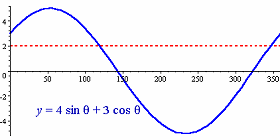We can see from the graph that in the domain 0° ≤ θ < 360°, the only two angles which give a value of 2 are 119.6° and 346.7°. So our answer is correct.

2. Solve the equation

sin theta-sqrt2 cos theta=0.8, for 0° ≤ θ < 360°.

Firstly, express the LHS in the form R sin(θα) (Note the negative sign!):

sin theta-sqrt2 cos theta R sin(theta-alpha)

R=sqrt(1^2+(sqrt2)^2)= sqrt(1+2)= sqrt3

alpha=arctan(sqrt2/1)=54.74^@

So

sin theta-sqrt2\ cos theta sqrt3\ sin(theta-54.74^@)

Now, solving the original equation gives:

sqrt3\ sin(theta-54.74^@)=0.8

sin(theta-54.74^@)=0.8/sqrt3=0.4619

Sine is positive in Quadrants I and II.

So, from calculator, we get

theta-54.74^@=27.51^@

OR

theta-54.74^@=152.49^@

And this gives us:

theta=27.51^@+54.74^@=82.25^@

OR

theta=152.49^@+54.74^@=207.23^@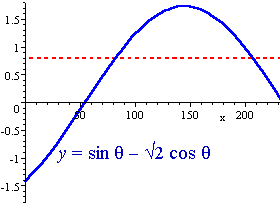We can see from the graph that in the domain 0^@ ≤ θ < 360^@, the only two angles which give a value of 0.8 (the red dotted line) are 82.3^@ and 207.2^@. So our answer is correct.

3. The current i (in amperes) at time t in a particular circuit is given by

i = 12 sin t + 5 cos t.

Find the maximum current and the first time that it occurs.

Note that normally we take t ≥ 0. For this sort of example, we must use radians for the angle.

We have:

R=sqrt(12^2+5^2)=13 and alpha=arctan(5/12)=0.39479.

So 12\ sin t+5\ cos t= 13\ sin(t+0.39479)

So we can see that the amplitude is 13 A and this is the maximum value.

To find the first time that it occurs, we need to solve

13\ sin(t+0.39479)=13

That is

sin(t+0.39479)=1

Now sin θ = 1 for the first time when theta=pi/2. So we need to solve:

t+0.39479=pi/2

t=pi/2-0.39479=1.176

So the maximum value of 13 A will first occur at time t = 1.176 s.

We can see that this is correct from the graph:

i=12\ sin t+5\ cos t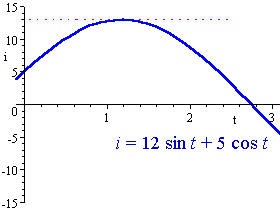4. Solve 7 sin 3θ − 6 cos 3θ = 3.8     for 0° ≤ θ < 360°.

Firstly, express the LHS in the form R sin(3θα).

(Note the negative sign and the 3θ! We have to increase the domain by 3 times.)

7\ sin 3theta-6\ cos 3theta R\ sin(3theta-alpha)

R=sqrt(7^2+6^2) =sqrt(49+36)=sqrt85

alpha=arctan(6/7)=40.60^@

So

7\ sin 3theta-6\ cos 3theta sqrt85sin(3theta-40.60^@)

Now, solving the original equation gives:

sqrt85sin(3theta-40.60^@) =3.8

 sin(3theta-40.60^@) =3.8/sqrt85 =0.412

Since we have 3θ , we must use the domain: 0^@ ≤ 3θ < 1080^@.

Sine is positive in Quadrants I,II and V, VI and IX and X.

So, from our calculator, we get the following for (3θ − 40.60^@):

24.33^@, 155.67^@, 384.33^@, 515.67^@, 744.33^@ and 875.67^@

So 3θ will be:

64.93^@, 196.27^@, 424.93^@, 556.27^@, 784.93^@, 916.27^@.

So the solutions for θ are:

21.6^@, 65.4^@, 141.6^@, 185.4^@, 261.6^@, 305.4^@.

5. The current i amperes in a certain circuit after t seconds is given by

i=2\ sin(t-pi/3)-cos(t+pi/2)

Find the maximum current and the earliest time it occurs.

(Note: t > 0)

We need to get this in a simpler form. In this one, notice that the angles in the brackets are not the same!

We must simplify them first so the angles in the brackets are the same.

i=2\ sin(t-pi/3)-cos(t+pi/2)

=2(sin t cos {:pi/3:}-cos t sin {:pi/3:} ) -(cos t cos {:pi/2:} - sin t sin {:pi/2:})

=2((sin t)/2-0.866\ cos t) -(cos txx0-sin t xx1)

=sin t-1.732\ cos t+sin t

=2\ sin t-1.732\ cos t

Now we can express i in the form R sin(t + α).

R=sqrt(2^2+1.732^2)=2.646

alpha=arctan(1.732/2)= 0.714\ text(radians

So

2\ sin t − 1.732\ cos t =  2.646\ sin(t − 0.714)

So the maximum value of this is 2.646\ "A".

To find when this occurs, we need to solve:

2.646\ sin(t − 0.714) = 2.646

i.e. sin(t − 0.714) = 1

t − 0.714 = π/2

t = 2.29

So t = 2.29\ "s" is the time when the maximum is first reached.

## The Cosine Form

We can also express our sum of a sine term and a cosine term using cosine rather than sine. It may be more convenient to do so in some situations.

The expressions obtained are similar to those we obtained for the sine case, but note the differences as we proceed.

For a, b and R positive and α acute, our equivalent expression is given by:

a sin θ + b cos θR cos (θ − α)

This time there is a difference in the way we obtain α, compared to before.

Expanding R cos (θ − α) using our result for the expansion of cos(A − B) gives us:

R cos (θ − α) = R cos θ cos α + R sin θ sin α

Rearranging and equating co-efficients gives us

a sin θ + b cos θ R cos α cos θ + R sin α sin θ

So:

a = R sin α ..... (1)

b = R cos α ..... (2)

Dividing (1) by (2) gives us:

a/b=(R sin alpha)/(R cos alpha)=tan alpha

Therefore:

alpha=arctan\ a/b

(Note the fraction is a/b for the "cosine" case, whereas it is b/a for the "sine" case.)

We find R the same as before:

R=sqrt(a^2+b^2)

So the sum of a sine term and cosine term have been combined into a single cosine term:

a sin θ + b cos θR cos(θ − α)

Once again, a, b, R and α are positive constants and α is acute.

## The Cosine Minus Case

If we have a sin θb cos θ and we need to express it in terms of a single cosine function, the formula we need to use is:

a sin θb cos θ ≡ −R cos (θ + α)

Once again, a, b and R are positive.

α is acute and given by:

alpha=arctan\ a/b

R is given by:

R=sqrt(a^2+b^2

### Cosine Exercises

1. Express 7 sin θ + 12 cos θ in the form R cos (θ − α), where 0 ≤ α < π/2.

We find α using

alpha=arctan\ a/b

α has to be in radians for this example, since we are told 0 ≤ α < π/2.

Since a = 7 and b = 12, we have:

α = arctan (7/12) = 0.528

We find R using

R=sqrt(a^2+b^2

So R=sqrt(7^2+12^2)=13.892

Therefore we can write:

7 sin θ + 12 cos θ = 13.892 cos (θ − 0.528)

To check our answer, we draw the graphs of both y = 7 sin θ + 12 cos θ and y = 13.892 cos (θ − 0.528). We see that they are exactly the same. (Only one is shown).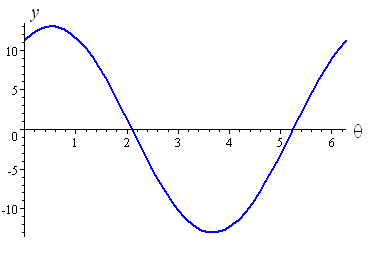We observe that our cosine graph has amplitude 13.892 and it has been shifted to the right by 0.528 radians, which is consistent with the expression we obtained: 13.892 cos (θ − 0.528)

2. Express 2.348 sin θ − 1.251 cos θ in the form −R cos (θ + α), where 0 ≤ α < π/2.

We find α using

a=text(arctan)a/b

Once again, α has to be in radians for this example.

Since a = 2.348 and b = 1.251, we have:

α = arctan (2.348/1.251) = 1.081

We find R using

R=sqrt(a^2+b^2)

So R=sqrt(2.348^2+ 1.251^2) = 2.660

So we can write:

2.340 sin θ − 1.251 cos θ = -2.660 cos (θ + 1.081)

Checking using a graph, we obtain the following for each side of our answer: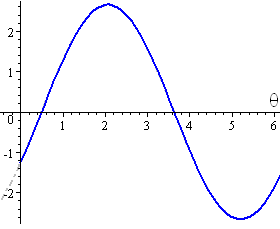We see that our negative cosine curve has an amplitude of 2.660 and it has been shifted to the left by 1.081 radians, which is consistent with the expression −2.660 cos (θ + 1.081).

## Summary

Here is a summary of the expressions and conditions that we have found in this section.

Original Expression Combined Expression α
a sin θ + b cos θ R sin (θ + α) alpha= arctan (b/a)
a sin θ b cos θ R sin (θα) alpha= arctan (b/a)
a sin θ + b cos θ R cos (θα) alpha= arctan (a/b)
a sin θ b cos θ −R cos (θ + α) alpha= arctan (a/b)

In each case, a, b and R are positive and α is an acute angle.

R is given by:

R=sqrt(a^2+b^2)

## Problem SolverThis tool combines the power of mathematical computation engine that excels at solving mathematical formulas with the power of GPT large language models to parse and generate natural language. This creates math problem solver thats more accurate than ChatGPT, more flexible than a calculator, and faster answers than a human tutor. Learn More.